Test: Pulse Modulation

# Test: Pulse Modulation - Electronics and Communication Engineering (ECE)

Test Description

## 10 Questions MCQ Test GATE ECE (Electronics) 2023 Mock Test Series - Test: Pulse Modulation

Test: Pulse Modulation for Electronics and Communication Engineering (ECE) 2023 is part of GATE ECE (Electronics) 2023 Mock Test Series preparation. The Test: Pulse Modulation questions and answers have been prepared according to the Electronics and Communication Engineering (ECE) exam syllabus.The Test: Pulse Modulation MCQs are made for Electronics and Communication Engineering (ECE) 2023 Exam. Find important definitions, questions, notes, meanings, examples, exercises, MCQs and online tests for Test: Pulse Modulation below.
Solutions of Test: Pulse Modulation questions in English are available as part of our GATE ECE (Electronics) 2023 Mock Test Series for Electronics and Communication Engineering (ECE) & Test: Pulse Modulation solutions in Hindi for GATE ECE (Electronics) 2023 Mock Test Series course. Download more important topics, notes, lectures and mock test series for Electronics and Communication Engineering (ECE) Exam by signing up for free. Attempt Test: Pulse Modulation | 10 questions in 30 minutes | Mock test for Electronics and Communication Engineering (ECE) preparation | Free important questions MCQ to study GATE ECE (Electronics) 2023 Mock Test Series for Electronics and Communication Engineering (ECE) Exam | Download free PDF with solutions
 1 Crore+ students have signed up on EduRev. Have you?
Test: Pulse Modulation - Question 1

### The Nyquist rate for a continuous-time signal (x) is x(t) = 6cos50πt + 20sin300πt - 10cos100πt

Detailed Solution for Test: Pulse Modulation - Question 1

Given,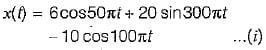Also,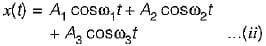Comparing equations (I) and (II), we have: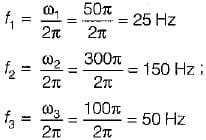Thus, the highest frequency component of the given message signal will be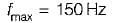∴ Nyquist rate = 2fmax = 2 x 150 = 300 Hz

Test: Pulse Modulation - Question 2

### Which of the following pulse modulation systems is analog?

Detailed Solution for Test: Pulse Modulation - Question 2

PAM, PWM and PPM are examples of analog pulse modulation techniques while PCM is a digital pulse modulation.

Test: Pulse Modulation - Question 3

### The Nyquist interval for the signal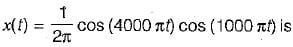Detailed Solution for Test: Pulse Modulation - Question 3

Given signal is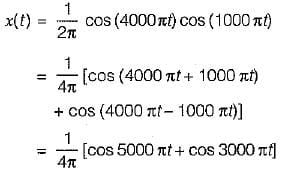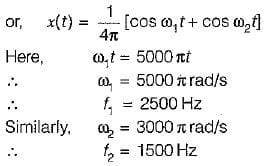Hence, the maximum frequency present in x(t) is f1= 2500 Hz
∴ Nyquist rate is given as
fs = 2fm
(where, fm = Maximum frequency present in the signal)
Here, fm = f1 = 2500 Hz
∴ Nyquist rate is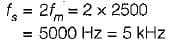Nyquist interval is given as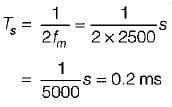Test: Pulse Modulation - Question 4

A telephone signal with cut-off frequency of 4 kHz is digitized into 8 bit PCM, sampled at Nyquist rate. The quantization S/N ratio is

Detailed Solution for Test: Pulse Modulation - Question 4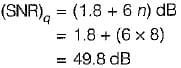Test: Pulse Modulation - Question 5

A sine wave of frequency fm and amplitude Am is applied to a delta modulator having step size Δ. The slope overload distortion will not occur if

Detailed Solution for Test: Pulse Modulation - Question 5

Let, x(t) = Am sin (2πfmt)
The slope of x(t) will be maximum when derivative of x(t) w.r.t. 't' will be maximum.
Maximum slope = Δ/Ts
Hence, for slope overload distortion not to occur,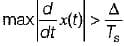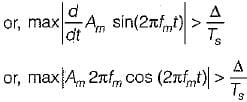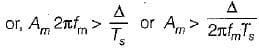Hence, slope overload distortion doesn’t occur if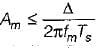Test: Pulse Modulation - Question 6

The output signal to noise ratio (SNR) of a 10-bit PCM was found to be 30 dB. The desired SNR is 42 dB. It was decided to increase the SNR to the desired value by increasing the number of quantization levels. The percentage increase in the transmission bandwidth required for this SNR is

Detailed Solution for Test: Pulse Modulation - Question 6

Given, SNR = 30 dB, n = 10,
Desired value of SNR = 42 dB
With the increase in n by 1 bit, the value of SNR increases by 6 dB. Therefore, to increase the value of SNR by 12 dB, it is necessary to increase n by 2,
∴ n = 10 + 2 = 12
Now, BW of PCM system = 1/2 nfs
Therefore, BW with n = 10 is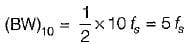and BW for n = 12 is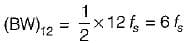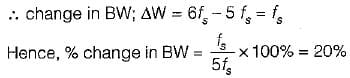Test: Pulse Modulation - Question 7

The biggest disadvantages of PCM is

Test: Pulse Modulation - Question 8

The bandwidth of TV video plus audio signal is 4.5 MHz. If this signal is converted into PCM bit stream with 1024 quantization levels and the signal is sampled at the rate 20% above Nyquist rate, then the number of bits/sec of the resulting signal is

Detailed Solution for Test: Pulse Modulation - Question 8

Given,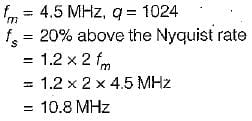Now, quantization level, q = 2n
(n = No. of bits/sec)
∴ 2n = 1024 = 210
or, n = 10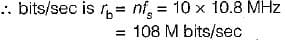Test: Pulse Modulation - Question 9

Assertion (A) : PCM has much better noise immunity as compared to PAM, PWM and PPM systems.
Reason (R) : PCM does not contain any information in the width or the position of the pulses.

Detailed Solution for Test: Pulse Modulation - Question 9

In practice, the transmitted pulses usually have slightly sloping sides (edges). As the noise is superimposed on them, the width and the position of the regenerated pulses is changed. This distorts the information contents in the PWM and PPM signals. Since PCM does not contain any information in the width or the position of the pulses, therefore PCM has much better noise immunity as compared to PAM, PWM and PPM systems. Hence, both assertion and reason are true and reason is the correct explanation of assertion.

Test: Pulse Modulation - Question 10

Assertion (A) : The signaling rate and transmission channel bandwidth is quite large for delta modulation compared to PCM.
Reason (R) : There is no analog to digital converter required in delta modulation unlike PCM.

Detailed Solution for Test: Pulse Modulation - Question 10

Since, the delta modulation transmits only one bit for one sample, therefore the signaling rate and transmission channel bandwidth is quite small for delta modulation compared to PCM, Hence, assertion is a false statement.
The transmission and receiver implementation is very simple for delta modulation since there is no A/D converter required in delta modulation unlike PCM. Hence, reason is a true statement.

## GATE ECE (Electronics) 2023 Mock Test Series

21 docs|263 tests
Information about Test: Pulse Modulation Page
In this test you can find the Exam questions for Test: Pulse Modulation solved & explained in the simplest way possible. Besides giving Questions and answers for Test: Pulse Modulation, EduRev gives you an ample number of Online tests for practice

## GATE ECE (Electronics) 2023 Mock Test Series

21 docs|263 tests(Scan QR code)